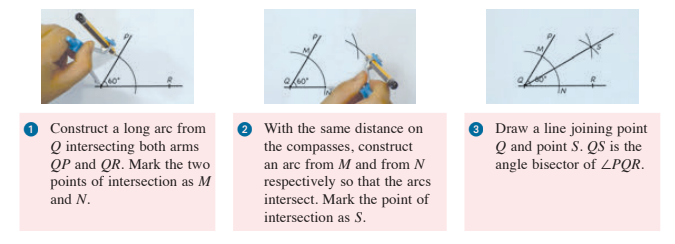## Lines and Angles

 8.1 Lines and Angles

Congruent line segments:

 Definition Line segments having the same length.

• A line segment is denoted using capital letters at both ends.

 Example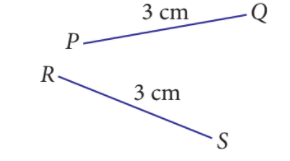We can see that both line segments, $$PQ$$ and $$RS$$, have the same length. Thus, $$PQ$$ and $$RS$$ are congruent.

Congruent angles:

 Definition Angles having the same size.

• An angle is denoted using the symbol ‘$$\angle$$’ and capital letters at the vertex and at the ends of the two arms of the angle.

 Example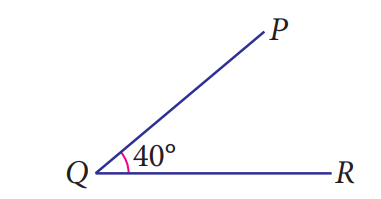We can see that, $$\angle PQR=40^\circ$$ or $$\angle RQP=40^\circ$$. Thus, $$\angle PQR$$ and $$\angle RQP$$ are congruent.

Estimate and measure the length of a line segment and the size of an angle:

• An angle that appears more than a right angle has an angle greater than $$90^\circ$$.
• An angle that appears less than a right angle has an angle less than $$90^\circ$$.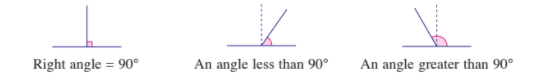• The size of an angle can be measured more accurately using a protractor.

The properties of the angle on a straight line, a reflex angle and the angle of one whole turn:

The angle on a straight line

• The sum of angles on a straight line is $$180^{\circ}$$.

Reflex angle

• An angle with a size more than $$180^{\circ}$$ and less than $$360^{\circ}$$.

The angle of one whole turn

• The sum of angles at a point is $$360^{\circ}$$.

The properties of complementary angles, supplementary angles and conjugate angles:

Complementary angles

• The sum of the two angles is always $$90^\circ$$.

Supplementary angles

• The sum of the two angles is always $$180^{\circ}$$.

Conjugate angles

• The sum of the two angles is always $$360^{\circ}$$.

Perform a geometrical construction:

(i) Line segments

• A section of a straight line with a fixed length.

 Example Construct the line segment $$AB$$ with a length of $$8\text{ cm}$$ using only a pair of compasses and a ruler.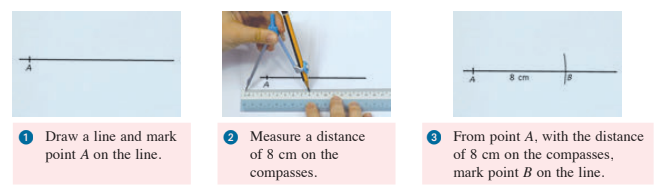(ii) Perpendicular bisectors

• If line $$AB$$ is perpendicular to line segment $$CD$$ and divide $$CD$$ into two parts of equal length, then line $$AB$$ is known as the perpendicular bisector of $$CD$$.

 Example Construct the perpendicular bisector of line segment $$PQ$$ using only a pair of compasses and a ruler.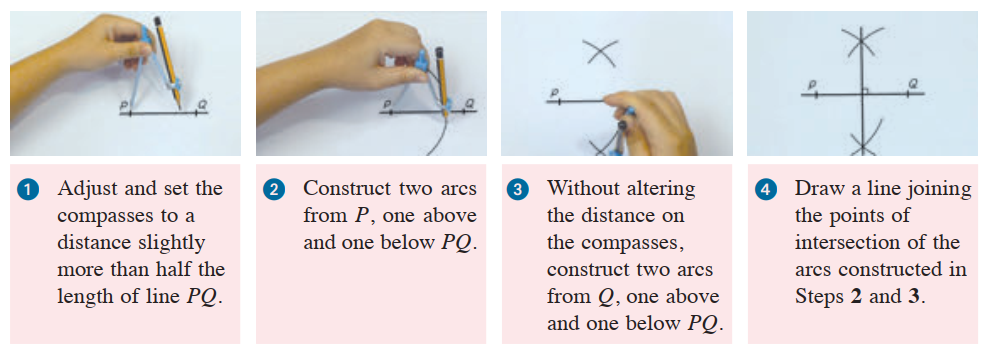(iii) Perpendicular line to straight line

• If a line is perpendicular to line $$PQ$$, then the line is known as perpendicular line to line $$PQ$$.

 Example Using only a pair of compasses and a ruler, construct the perpendicular line from point $$M$$ to the straight line $$PQ$$.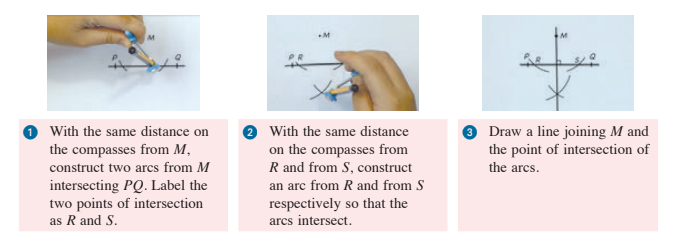(iv) Parallel lines

• Lines that will never meet even when they are extended.

 Example Using only a pair of compasses and a ruler, construct the line that is parallel to $$PQ$$ passing through point $$R$$.Construct angles and angle bisectors:

(i) Constructing an angle of $$60^{\circ}$$

 Example Using only a pair of compasses and a ruler, construct line $$PQ$$ so that $$\angle PQR=60^\circ$$.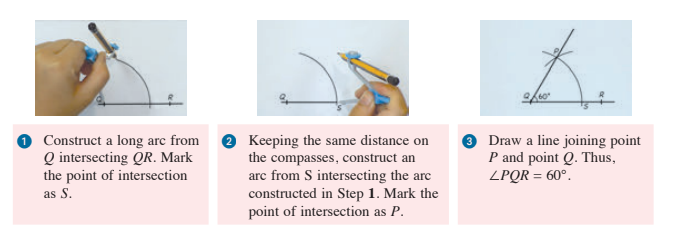(ii) Angle Bisectors

• A line divides an angle into two equal angles.

 Example Using only a pair of compasses and a ruler, construct the angle bisector of $$\angle PQR$$.